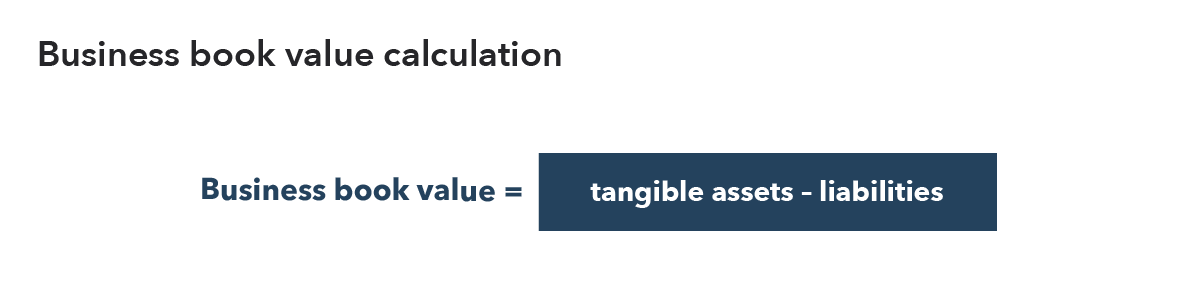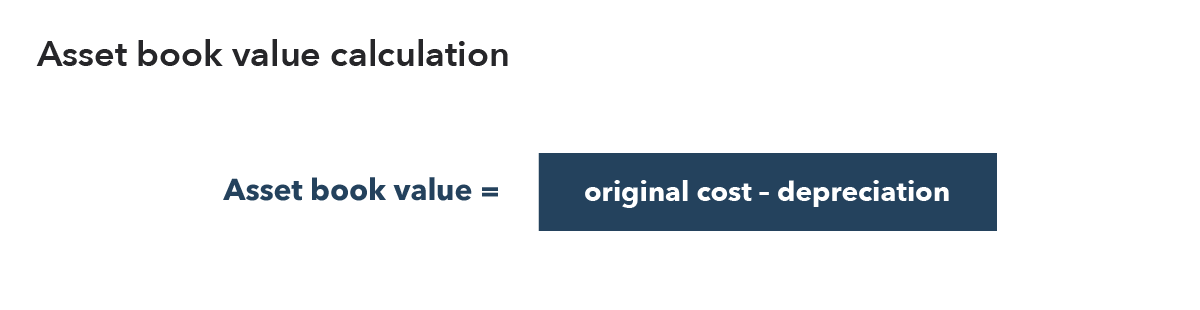Spread bets and CFDs are complex instruments and come with a high risk of losing money rapidly due to leverage. 76% of retail investor accounts lose money when trading spread bets and CFDs with this provider. You should consider whether you understand how spread bets and CFDs work, and whether you can afford to take the high risk of losing your money.
Spread bets and CFDs are complex instruments and come with a high risk of losing money rapidly due to leverage. 76% of retail investor accounts lose money when trading spread bets and CFDs with this provider. You should consider whether you understand how spread bets and CFDs work, and whether you can afford to take the high risk of losing your money.

# Book value definition

## What is book value?

Book value, or net book value, is the term used to describe how much a business or asset is worth according to its financials. For businesses, it is the total value of tangible assets minus liabilities. And for assets, it is the amount that was paid for the asset minus depreciation – which is the decline in an asset’s value due to market conditions.### Difference between book value and market value

While book value reflects what a business is worth according to its financials (its books), market value is the worth of a company according to financial markets – also known as its market capitalisation. The calculation for market value is the current market price per share multiplied by the total number of outstanding shares.

## Learn how to trade stocks

Go long or short on over 13,000+ international shares with CFDs or spread betting.

## Book value example

To calculate the book value of a company, you would use the total amount of tangible assets and subtract the liabilities. For example, ABC Limited has £320 million in assets and £190 million in liabilities. In this case, the company’s book value will be £130 million (£320 million – £190 million).

To determine the book value per share in the company, you would divide the book value by the number of shares in circulation. For example, £130 million divided by 500,000 shares = £260.

## How do traders and investors use book value?

Book value is used by traders and investors to compare different companies, and to find undervalued or overvalued stocks. This is done by using the price-to-book (P/B) ratio, which assesses a company’s current market price against its book value.

To calculate the P/B ratio, you would simply divide the market price per share by the book value per share. Shares would be considered undervalued if the P/B ratio is lower than one, and overvalued if it’s significantly more than one.

## Pros and cons of book value

### Pros of book value

Using the book value calculation can show how much a business or asset is worth based on data, rather than on speculation or opinion. Therefore, it is considered a relatively accurate measure of value. This means it can be useful when trying to learn more about a company or find stocks at a fair price.

### Cons of book value

Book value alone is not a definitive measure of value. This is because book value is ineffective at valuing intangible assets, such as intellectual property rights. For example, companies that develop software might be able to develop products for a relatively low cost, so the balance sheet might not reflect the true value of the assets. This could mean that the company’s shares trade at many times their book value but should not be considered overvalued.

Book value should therefore be used as a comparative measure (to compare assets and companies with one another).

Discover how to trade with IG Academy, using our series of interactive courses, webinars and seminars.

A - B - C - D - E - F - G - H - I - J - K - L - M - N - O - P - Q - R - S - T - U - V - W - Y